Question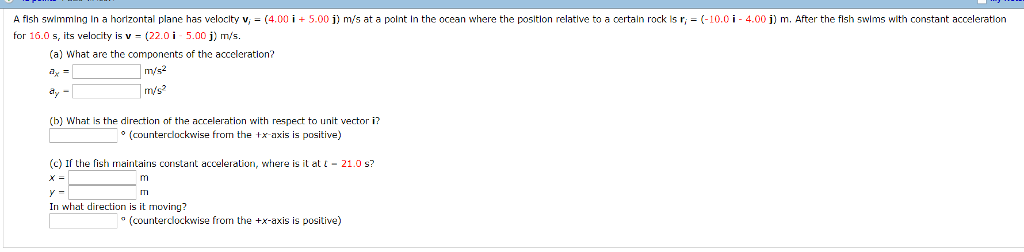We know that

V= Vi+at

Where V=final velocity

Vi=initial velocity

a= constant acceleration

t= time

Let a=axi+ ay​​​​​​j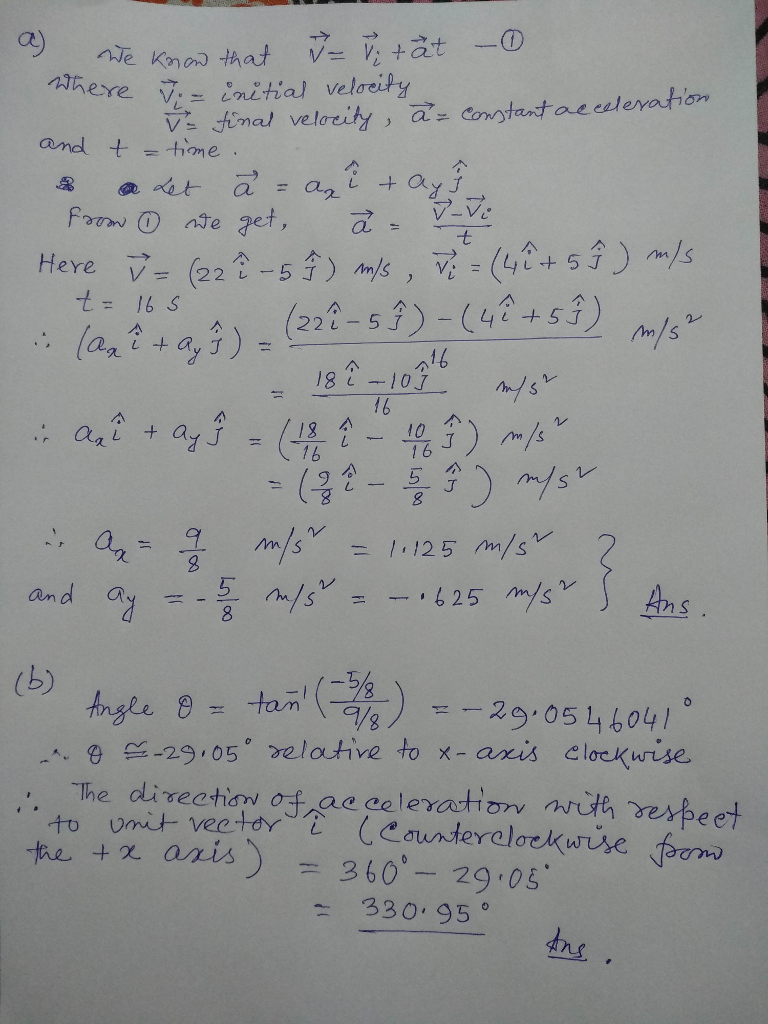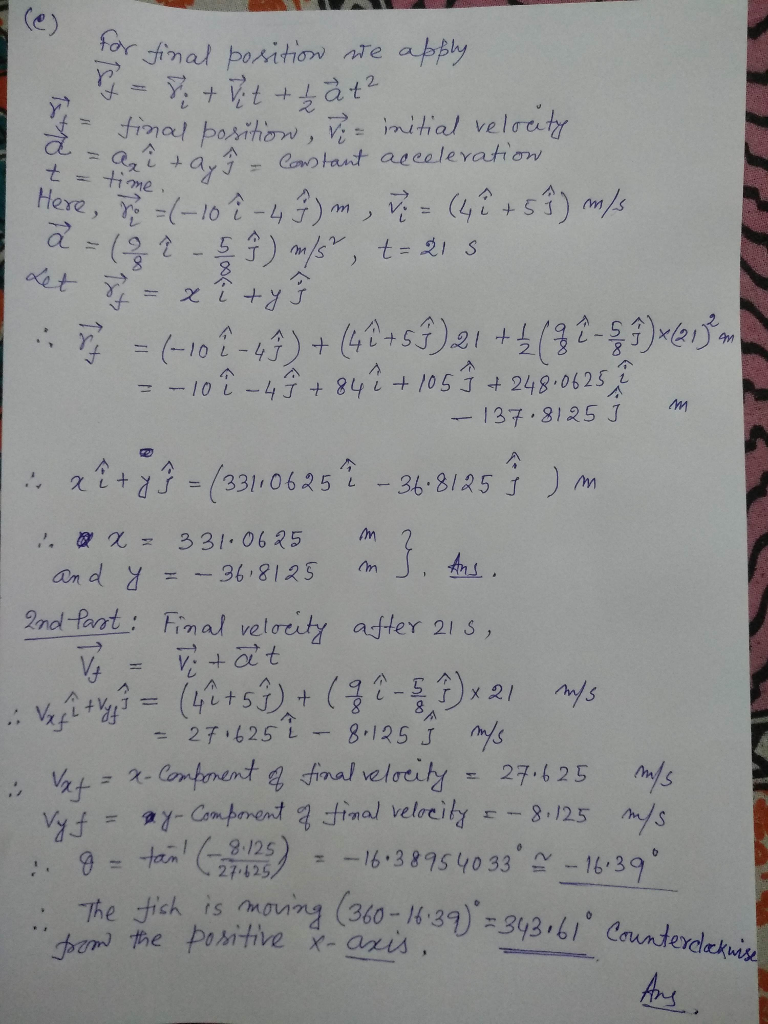​​​​​​

#### Earn Coins

Coins can be redeemed for fabulous gifts.

Similar Homework Help Questions
• ### A fish swimming in a horizontal plane has velocity v.- 4.00 + 1.00 j m s...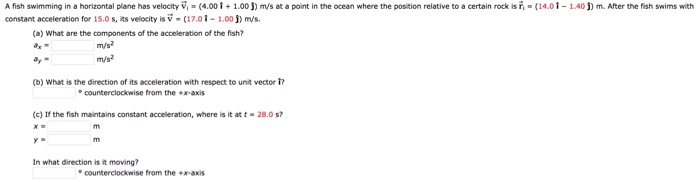A fish swimming in a horizontal plane has velocity v.- 4.00 + 1.00 j m s at a point in the ocean where the post on relative to a certain rock sn· constant acceleration for 15.0 s, its velocity is v (01 1.00j) m/s. 14,0 1-140 m. After the sh swims wit (a) What are the components of the acceleration of the fish? ay (b) What is the direction of its acceleration with respect to unit vector ? ecounterclockwise from...

• ### Please help! A fish swimming in a horizontal plane has velocity v = (4.00 i +...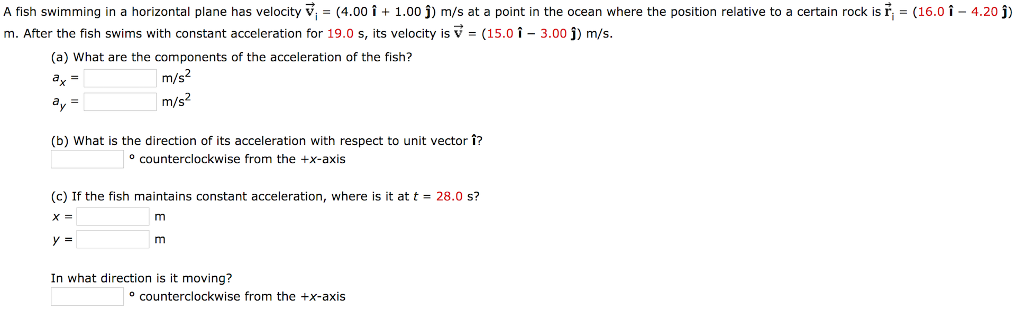Please help! A fish swimming in a horizontal plane has velocity v = (4.00 i + 1.00 j) m/s at a point in the ocean where the position relative to a certain rock is ri = (16.0-4.20 j) m. After the fish swims with constant acceleration for 19.0 s, its velocity is V (15.0 1-3.00 j) m/s. (a) What are the components of the acceleration of the fish? m/s m/s (b) What is the direction of its acceleration with respect...

• ### Help! A fish swimming in a horizontal plane has velocity V (4.00 i1.00 j) m/s at...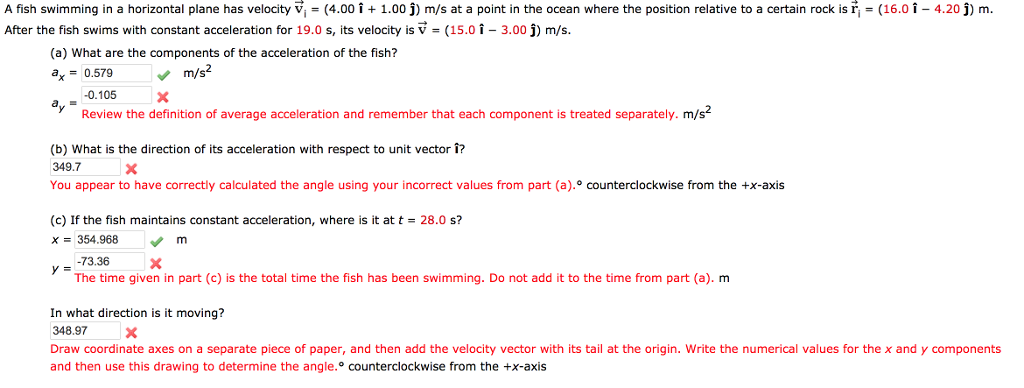Help! A fish swimming in a horizontal plane has velocity V (4.00 i1.00 j) m/s at a point in the ocean where the position relative to a certain rock is fter the fish swims with constant acceleration for 19.0 s, its velocity is V (15.0 1-3.00 j) m/s (16.0 1 4.20) m (a) What are the components of the acceleration of the fish? ax0.579 m/s2 0.105X Review the definition of average acceleration and remember that each component is treated separately....

• ### A fish swimming in a horizontal plane has velocity v with arrowi = (4.00 i +...

A fish swimming in a horizontal plane has velocity v with arrowi = (4.00 i + 1.00 j) m/s at a point in the ocean where the position relative to a certain rock is r with arrowi = (14.0 i − 4.20 j) m. After the fish swims with constant acceleration for 19.0 s, its velocity is v with arrow = (15.0 i − 3.00 j) m/s. (a) What are the components of the acceleration of the fish? ax =...

• ### A fish swimming in a horizontal plane has velocity m/s at a point in a the...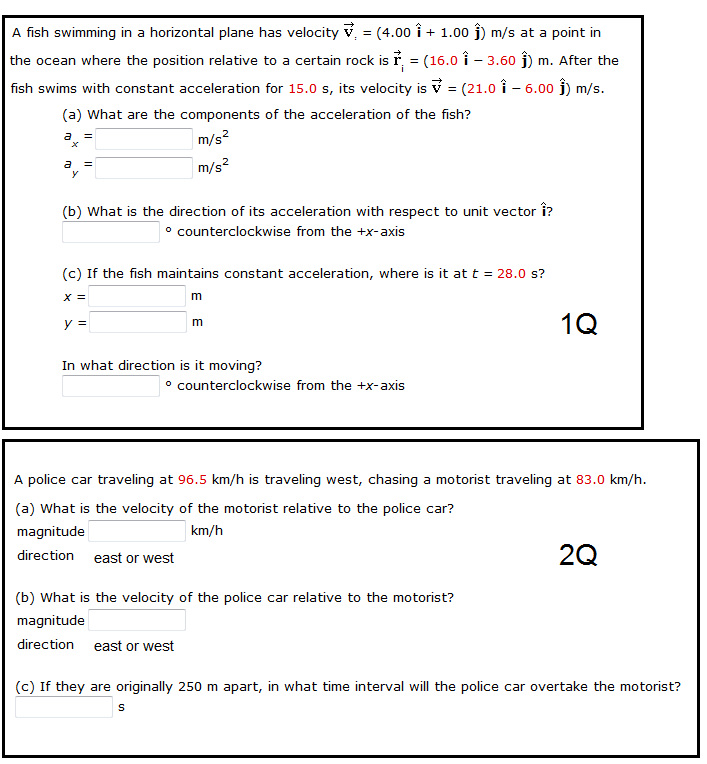A fish swimming in a horizontal plane has velocity m/s at a point in a the ocean where the position relative to a certain rock is m. After the fish swims with constant acceleration for 15.0 s, its velocity is m/s. What are the components of the acceleration of the fish? What is the direction of its acceleration with respect to unit vector ? counterclockwise from the +x-axis If the fish maintains constant acceleration, where is it at t =...

• ### just the last one idk why its wrong A fish swimming in a horizontal plane has...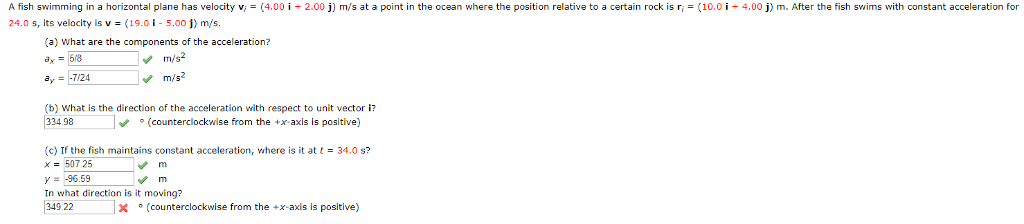just the last one idk why its wrong A fish swimming in a horizontal plane has velocity v 24.0 s, its velocity is v(19.01-5.001) m/s 4.00 і 2.00 j) m s at a point in the ocean where the position relat ve to a certan rocks 4.00 m After the ist swi snitt constan acceleration (a) What are the components of the acceleration? a,-58 m/s2 (b) what is the direction of the acceleration with respect to unit vector i 334...

• ### If the number is a decimal please include 4 digits past the decimal point if available....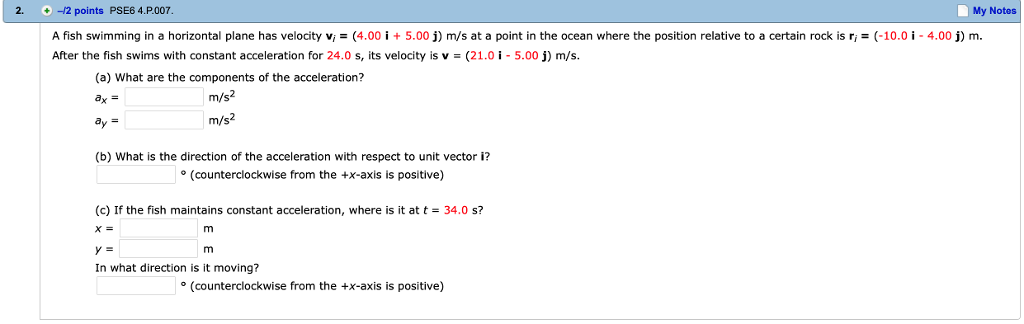If the number is a decimal please include 4 digits past the decimal point if available. 2. -12 points PSE6 4.P.007 My Notes A fish swimming in a horizontal plane has velocity vi = (4.00 İ + 5.00 j) m/s at a point in the ocean where the position relative to a certain rock is ri = (-10.01-4.00 j) m. After the fish swims with constant acceleration for 24.0 s, its velocity is v-(21.0-5.00) m/s. (a) What are the components...

• ### 2O6 points SerPSE9 4.P.009 MLFB My Notes Ask Your h swimming in a horizontal plane has...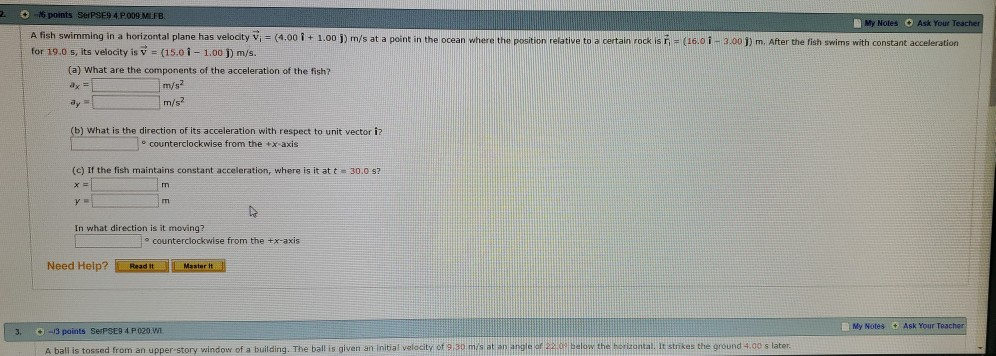2O6 points SerPSE9 4.P.009 MLFB My Notes Ask Your h swimming in a horizontal plane has velocity Vi (4.00 i + 1.00 j) m/s at a point in the ocean where the position relative to a certain rock isi- (16.01 -3.00 1) m. After the fish swims with constant acceleration for 19.0 s, its velocity is v (15.01-1.00 j) m/s. (a) What are the components of the acceleration of the fish? m/s2 m/s2 b) What is the direction of its...

• ### 5. + 0/1 points Previous Answers SerPSE 10 4.2.OP.005.MI. My Notes A fish swimming in a...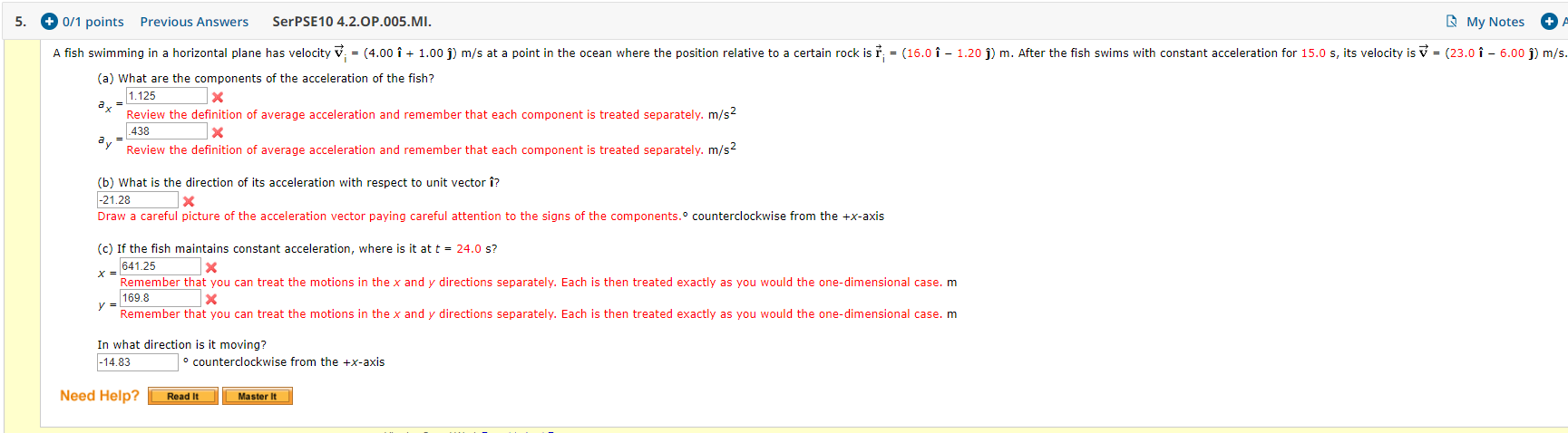5. + 0/1 points Previous Answers SerPSE 10 4.2.OP.005.MI. My Notes A fish swimming in a horizontal plane has velocity v = (4.00 i + 1.00 )) m/s at a point in the ocean where the position relative to a certain rock is † ; = (16.0 î - 1.20 j) m. After the fish swims with constant acceleration for 15.0 s, its velocity is v = (23.0 î - 6.00 ) m/s. (a) What are the components of the...

• ### Please answer all. The displacement vectors A and B shown in the figure below both have...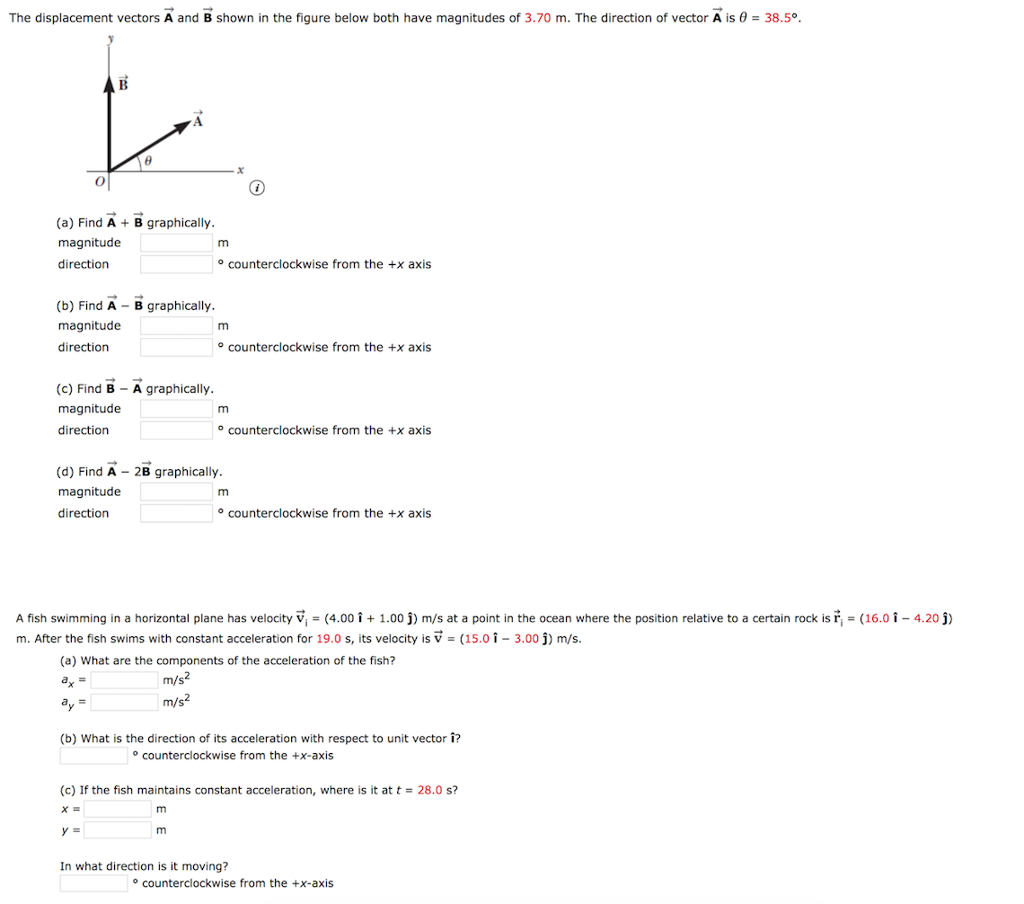Please answer all. The displacement vectors A and B shown in the figure below both have magnitudes of 3.70 m. The direction of vector A is θ = 38.5° (a) Find A B graphically magnitude direction o counterclockwise from the +x axis (b) Find A B graphically magnitude direction o counterclockwise from the +x axis (c) Find B A graphically magnitude direction o counterclockwise from the +x axis (d) Find A 2B graphically magnitude direction o counterclockwise from the +x...